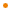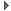# JavaFX: Bringing Rich Experiences To All the Screens Of Your Life

### Overview

A class that defines a duration of time. Duration instances are defined in milliseconds, but can be easily created using time literals; for example, a two-and-a-half minute Duration instance can be defined in several ways:

``````    Duration t = 2m + 30s;
Duration t = 2.5m;
Duration t = 2500ms;``````
Duration instances are immutable, and are therefore replaced rather than modified. To create a new Duration instance, either use a time literal, or use the `Duration.valueOf(milliseconds)` factory method.

Profile: common

### Script Variable Summary

accessnametypedescription
publicINDEFINITEDurationUsed to specify a duration of indefinite length.

### Script Function Summary

public valueOf(ms: Double) : Duration

Factory method that returns a Duration instance for a specified number of milliseconds.Factory method that returns a Duration instance for a specified number of milliseconds.

Parameters
ms
the number of milliseconds
Returns
Duration
a Duration instance of the specified number of milliseconds

Profile: common

&nbsp;
public valueOf(ms: Number) : DurationParameters
ms
Returns
Duration
&nbsp;

### Function Summary

public add(other: Duration) : Duration

Add this instance and another Duration instance to return a new Duration instance.Add this instance and another Duration instance to return a new Duration instance. If either instance is INDEFINITE, return INDEFINITE. This function does not change the value of the called Duration instance.

Parameters
other
Returns
Duration

Profile: common

&nbsp;
public div(n: Number) : Duration

Divide this instance by a number to return a new Duration instance.Divide this instance by a number to return a new Duration instance. If the called Duration instance is INDEFINITE, return INDEFINITE. This function does not change the value of the called Duration instance.

Parameters
n
Returns
Duration

Profile: common

&nbsp;
public div(other: Duration) : Number

Divide this instance by another Duration to return the ratio.Divide this instance by another Duration to return the ratio. If both instances are INDEFINITE, return NaN. If this instance is INDEFINITE, return POSITIVE_INFINITY If the other instance is INDEFINITE, return 0.0. This function does not change the value of the called Duration instance.

Parameters
other
Returns
Number

Profile: common

&nbsp;
public ge(other: Duration) : Boolean

Returns true if the specified duration is greater than or equal to (>=) this instance.Returns true if the specified duration is greater than or equal to (>=) this instance. INDEFINITE is treated as if it were positive infinity.

Parameters
other
Returns
Boolean

Profile: common

&nbsp;
public gt(other: Duration) : Boolean

Returns true if the specified duration is greater than (>) this instance.Returns true if the specified duration is greater than (>) this instance. INDEFINITE is treated as if it were positive infinity.

Parameters
other
Returns
Boolean

Profile: common

&nbsp;
public le(other: Duration) : Boolean

Returns true if the specified duration is less than or equal to (<=) this instance.Returns true if the specified duration is less than or equal to (<=) this instance. INDEFINITE is treated as if it were positive infinity.

Parameters
other
Returns
Boolean

Profile: common

&nbsp;
public lt(other: Duration) : Boolean

Returns true if the specified duration is less than (<) this instance.Returns true if the specified duration is less than (<) this instance. INDEFINITE is treated as if it were positive infinity.

Parameters
other
Returns
Boolean

Profile: common

&nbsp;
public mul(n: Number) : Duration

Multiply this instance with a number to return a new Duration instance.Multiply this instance with a number to return a new Duration instance. If the called Duration instance is INDEFINITE, return INDEFINITE. This function does not change the value of the called Duration instance.

Parameters
n
Returns
Duration

Profile: common

&nbsp;
public negate() : Duration

Return a new Duration instance which has a negative number of milliseconds from this instance.Return a new Duration instance which has a negative number of milliseconds from this instance. For example, `(50ms).negate()` returns a Duration of -50 milliseconds. If the called Duration instance is INDEFINITE, return INDEFINITE. This function does not change the value of the called Duration instance.

Returns
Duration

Profile: common

&nbsp;
public sub(other: Duration) : Duration

Subtract other Duration instance from this instance to return a new Duration instance.Subtract other Duration instance from this instance to return a new Duration instance. If either instance is INDEFINITE, return INDEFINITE. This function does not change the value of the called Duration instance.

Parameters
other
Returns
Duration

Profile: common

&nbsp;
public toHours() : Number

Returns the number of whole hours in this period or Number.POSITIVE_INFINITY if the period is INDEFINITE.Returns the number of whole hours in this period or Number.POSITIVE_INFINITY if the period is INDEFINITE.

Returns
Number

Profile: common

&nbsp;
public toMillis() : Double

Returns the number of milliseconds in this period or Double.POSITIVE_INFINITY if the period is INDEFINITE.Returns the number of milliseconds in this period or Double.POSITIVE_INFINITY if the period is INDEFINITE.

Returns
Double

Profile: common

&nbsp;
public toMinutes() : Number

Returns the number of whole minutes in this period or Number.POSITIVE_INFINITY if the period is INDEFINITE.Returns the number of whole minutes in this period or Number.POSITIVE_INFINITY if the period is INDEFINITE.

Returns
Number

Profile: common

&nbsp;
public toSeconds() : Number

Returns the number of whole seconds in this period or Number.POSITIVE_INFINITY if the period is INDEFINITE.Returns the number of whole seconds in this period or Number.POSITIVE_INFINITY if the period is INDEFINITE.

Returns
Number

Profile: common

&nbsp;

### Inherited Functions

Spec-Zone.ru - all specs in one place